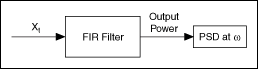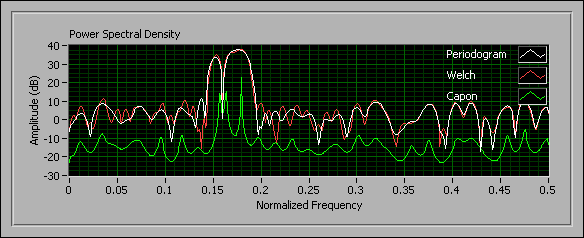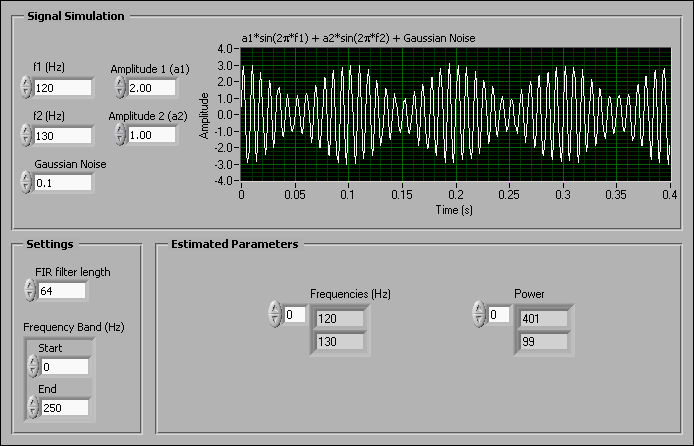# Using the Capon Method (Advanced Signal Processing Toolkit)

LabVIEW 2014 Advanced Signal Processing Toolkit Help

Edition Date: June 2014

Part Number: 372656C-01

»View Product InfoDownload Help (Windows Only)

The Capon method estimates the power spectral density (PSD) of a time series, X t, by using the output power of a finite impulse response (FIR) bandpass filter. The Capon method designs an FIR filter that suppresses all frequencies of an input signal except the frequency components at frequency ω. The following figure shows the Capon estimation method.The Capon method has a higher frequency resolution than the periodogram and Welch methods. Therefore, you can identify peaks more accurately with the Capon method than with these methods.

The following figure shows the PSDs based on the periodogram, Welch, and Capon methods.The PSD in green uses the Capon method. The PSDs in white and red use the Welch and periodogram methods, respectively. Notice that the peaks of the Capon method PSD are sharper.

The Capon method does not require prior knowledge about the input signal. Therefore, this method is less restrictive than model-based methods, such as the AR and ARMA methods, which require you to determine the model order before estimating the PSD.

You also can use the Capon method to determine the leading frequency and corresponding power magnitudes of an input signal. The following figure shows an example of a VI that uses the Capon method to estimate the leading frequency in a synthesized time series consisting of exponentially-damped sinusoids.In the Estimated Parameters section of the previous figure, notice that the Power at 120.0 Hz is greater than the Power at 130.0 Hz. Therefore, the leading frequency of this time series is 120.0 Hz.

Use the TSA Capon Spectrum VI to compute the PSD of a time series by using the Capon method. Use the TSA Capon Frequency Estimator VI to determine the leading frequency of a time series by using the Capon method.

Refer to the Power Spectral Density Estimation VI in the labview\examples\Time Series Analysis\TSAGettingStarted directory for an example that demonstrates how to estimate the PSD of a univariate time series by using different methods.

Refer to the Leading Frequency Estimator VI in the labview\examples\Time Series Analysis\TSAGettingStarted directory for an example that demonstrates how to extract the leading frequency components in exponentially-damped sinusoids by using the TSA Capon Frequency Estimator VI.